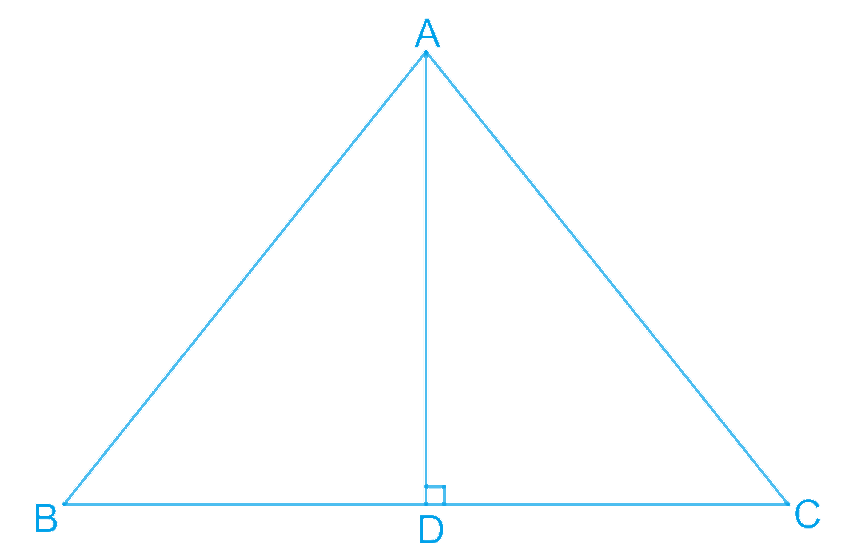# Ex.7.3 Q2 Triangles Solution - NCERT Maths Class 9

Go back to  'Ex.7.3'

## Question

$$AD$$ is an altitude of an isosceles triangle

$$ABC$$ in which $$AB = AC.$$ Show that

(i) $$AD$$ bisects $$BC$$

(ii) $$AD$$ bisects $$\angle A$$.

Video Solution
Triangles
Ex 7.3 | Question 2

## Text Solution

What is Known?

AD is an altitude of an isosceles triangle $$ABC$$ in which $$AB\,\, AC.$$

To prove:

i) $$AD$$ bisects $$BC$$

ii) $$AD$$ bisects

Reasoning:

We can show triangle $$BAD$$ and $$CAD$$ congruent by using RHS congruency criterion and then we can say corresponding parts of congruent triangle are equal.

Steps:(i) In $$\Delta BAD$$ and $$\Delta CAD$$,

\begin{align} \angle ADB &= \angle ADC\\text{Each }90^{\circ}&\text{ as }AD\text{ is the altitude})\\\\AB &= AC\;(\text{Given})\\AD &= AD\;(\text{Common})\\ \therefore \Delta BAD &\cong \Delta CAD\\(\text{By RHS }&\text{ Congruence rule})\end{align} \(\therefore BD = CD (By $$CPCT)$$

Hence, $$AD$$ bisects $$BC$$.

(ii) Also, by $$CPCT$$,

$$\angle BAD = \angle CAD$$

Hence, $$AD$$ bisects $$\angle A$$.

Video Solution
Triangles
Ex 7.3 | Question 2

Learn from the best math teachers and top your exams

• Live one on one classroom and doubt clearing
• Practice worksheets in and after class for conceptual clarity
• Personalized curriculum to keep up with school